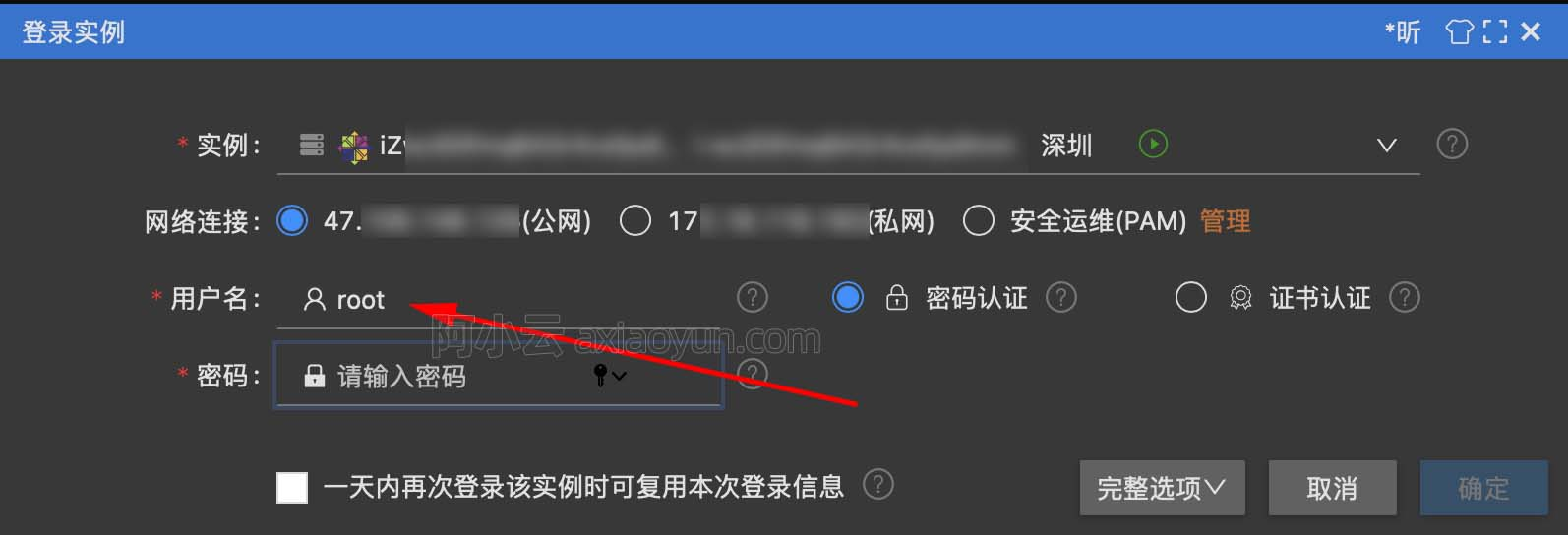# 贫下中农版jQuery

+关注继续查看

### 之前的代码

(function () {
var _NS = function () {

}

_NS.prototype.select = function (selector,context) {
var context = context || document;
return context.querySelectorAll(selector);
}

_NS.prototype.isArrayLike=function(obj){
if(obj instanceof Array){
return true;
}

var length=obj.length;
if ( obj.nodeType === 1 && length ) {
return true;
}
return false;
}

_NS.prototype.html = function (obj,value) {
var isArray=this.isArrayLike(obj), i=0;

if (typeof value == 'string') {
if (!isArray) {
obj.innerHTML = value;
} else {
var length = obj.length;
while (i < length) {
obj[i].innerHTML = value;
i += 1;
}
}
} else {
if (!isArray) {
return obj.innerHTML;
} else {
return obj.innerHTML;
}
}
}

window.NS = new _NS();
})();

var divs=NS.select('div');
divs.html('test');
divs.css('display','none');

### 为什么jQuery很方便

jQuery好用有几个原因：

1. $本身是个function对象，包含一些“静态方法”（不用实例化就可以用的方法），比如$.ajax、$.animation,可以这样$.xxx()直接使用jQuery的一些库函数

2. 因为$本身是一个函数，可以被调用。但是$(selector) 返回结果并不是搜索的结果集，而是一个jQuery实例，结果集被封装在jQuery对象内，这样可以使用一些jQUery的实例方法（也就是定义在prototype内的方法等），例如$(‘div’).html(‘test’), 这样由于$(‘div’)返回的是jQuery实例，所以可以调用实例方法html()。

3. jQuery对象大部分实例方法尽量返回jQuery对象，即调用者本身，这样可以支持链式调用，比如$(‘div’).html(‘test’).css(‘display’,’none’) ,$(‘div’)返回jQuery对象，里面包含结果集，调用实例方法html(‘test’) 同样返回jQuery对象，调用 css(‘display’,’none’) 同样也返回jQuery对象，可以这样一直调用下去。

### 构造函数的一些知识

1.什么样的函数是构造函数

2.构造函数返回什么样的结果

function A(){
return true;
}

var a=new A();
console.log(a instanceof A); //true

function B(){
return new Array();
}

var b=new B();
console.log(b instanceof Array); //true

### 做个贫下中农版的jQuery

};

$.ajax=function(configs){ //静态方法 //TODO }$.prototype.html=function(value){ //实例方法
//TODO
}

window.$=$;
})();

var context = context || document;
var nodeList = context.querySelectorAll(selector);

var $=new$();
$.elements=nodeList; return$;
};

$.ajax=function(configs){ //静态方法 //TODO }$.prototype.html=function(value){ //实例方法
//TODO
}

window.$=$;
})();

return f;
})();
window.$=$;
})();

version 0.4

(function(){
var $=(function(){ function f(selector,context){ return f.prototype.init(selector,context); } f.prototype.init=function(selector,context){ var context = context || document; var nodeList = context.querySelectorAll(selector); this.length = nodeList.length; this.elements=new Array(); for (var i = 0; i < this.length; i++) { this.elements[i] = nodeList[i]; } return this; } return f; })(); window.$=$; })(); 填上刚才自定义的函数 version 1.0 (function(){ var$=(function(){
function f(selector,context){
return f.prototype.init(selector,context);
}

f.ajax=function(configs){
//TODO
}

f.prototype.init=function(selector,context){
var context = context || document;
var nodeList = context.querySelectorAll(selector);
this.length = nodeList.length;
this.elements=new Array();
for (var i = 0; i < this.length; i++) {
this.elements[i] = nodeList[i];
}
,                        return this;
}

f.prototype.html=function(value){
//TODO
}

return f;
})();

window.$=$;
})();

### jQuery源码结构

(function( window, undefined ) {

var jQuery = (function() {
// 构建jQuery对象
var jQuery = function( selector, context ) {
return new jQuery.fn.init( selector, context, rootjQuery );
}

// jQuery对象原型
jQuery.fn = jQuery.prototype = {
constructor: jQuery,
init: function( selector, context, rootjQuery ) {
// selector有以下7种分支情况：
// DOM元素
// body（优化）
// 字符串：HTML标签、HTML字符串、#id、选择器表达式
// 最后返回伪数组
}
};

//把jQuery的prototype赋值给init方法的prototype
jQuery.fn.init.prototype = jQuery.fn;

// 合并内容到第一个参数中，后续大部分功能都通过该函数扩展
// 通过jQuery.fn.extend扩展的函数，大部分都会调用通过jQuery.extend扩展的同名函数
jQuery.extend = jQuery.fn.extend = function() {};

// 在jQuery上扩展静态方法
jQuery.extend({
// isPlainObject isEmptyObject
// parseJSON parseXML
// globalEval
// each makeArray inArray merge grep map
// proxy
// access
// uaMatch
// sub
// browser
});

return jQuery;

})();

window.jQuery = window.$= jQuery; })(window); 总体上是一致的，但是jQuery的结构要科学很多 1.将window对象传入匿名函数，使匿名函数内部可以直接访问，防止匿名函数内部使用window对象的时候需要层层查找作用域链，最后才能找到window 2. 没有一棍子打死，完全使用$，当出现$命名冲突的时候可以使用jQuery代替 3. 定义jQuery.fn=jQuery.prototype，代码写起来方便了很多，也有利于压缩 4. 没有使用elements属性，而是利用数组特性封装搜索结果集，在使用的时候更容易想到 5. 定义each函数用于遍历结果集 6. 提供extend函数用于向对象内部添加属性 ### 穷人版jQuery Version2.0 看了大师的写法终于可以脱离贫下中农了 (function () { var$ = (function () {
var $= function (selector, context) { return new$.prototype.init(selector, context);
}

$.prototype.init = function (selector, context) { var context = context || document; var nodeList = context.querySelectorAll(selector); this.length = nodeList.length; for (var i = 0; i < this.length; i++) { this[i] = nodeList[i]; } return this; }$.prototype.each = function (callback, args) {
var length = this.length, i = 0;
if (args) {
while (i < length) {
callback.call(this[i], args);
i += 1;
}
} else {
while (i < length) {
callback.call(this[i]);
i += 1;
}
}
return this;
}

$.prototype.html = function (value) { if (typeof value == 'string') { this.each(function () { this.innerHTML = value; }); return this; } else { return this.innerHTML; } }$.prototype.init.prototype = $.prototype; return$;
})();

window.$=$;
})();

### 最后

本文转自魏琼东博客园博客，原文链接：http://www.cnblogs.com/dolphinX/p/3270779.html，如需转载请自行联系原作者19773 019337 029075 063949 022531 0Xshell使用SSH远程登录阿里云ECS服务器CentOS7
22308 020686 016420 014896 023576 0
3402

0

JS零基础入门教程（上册）